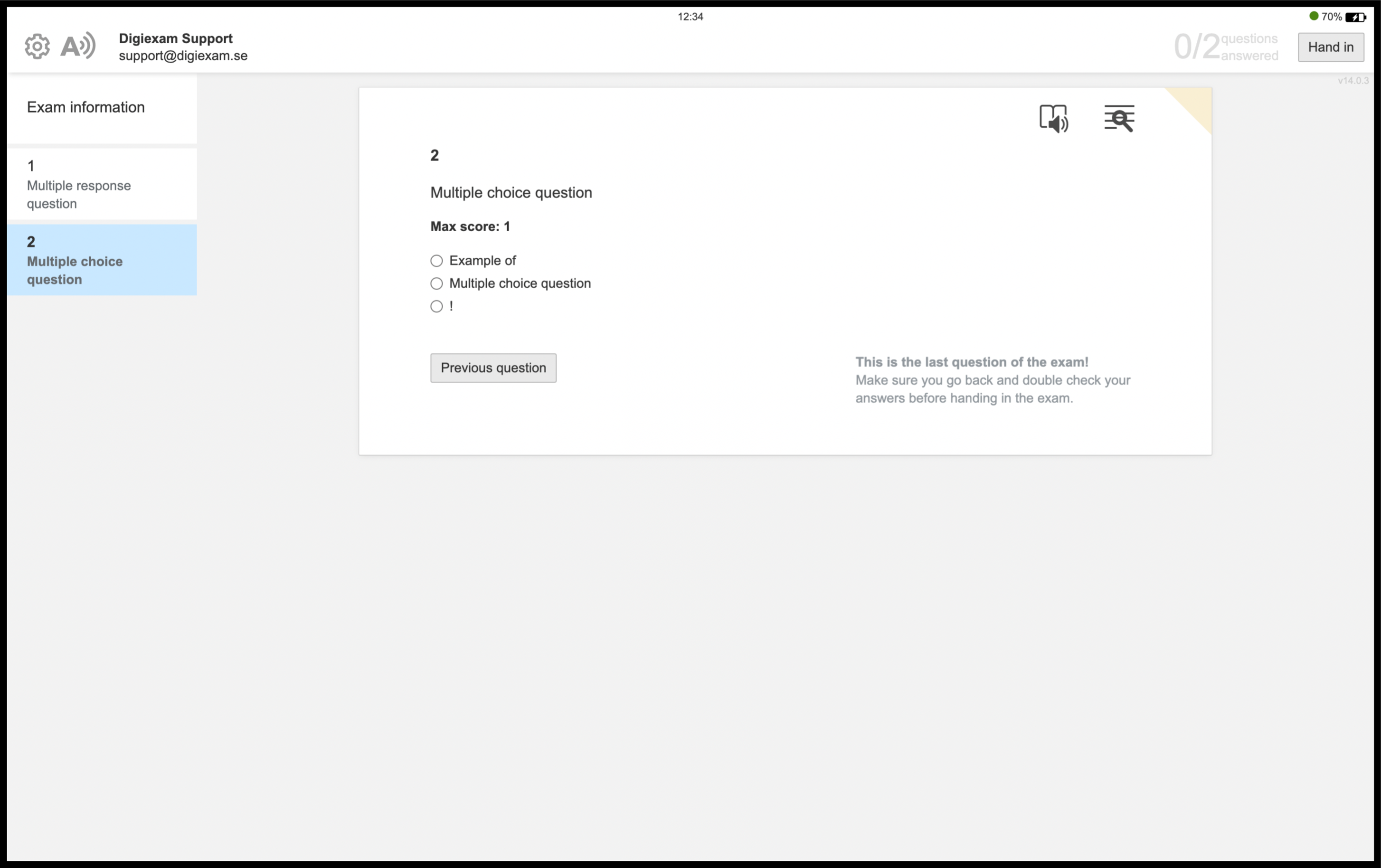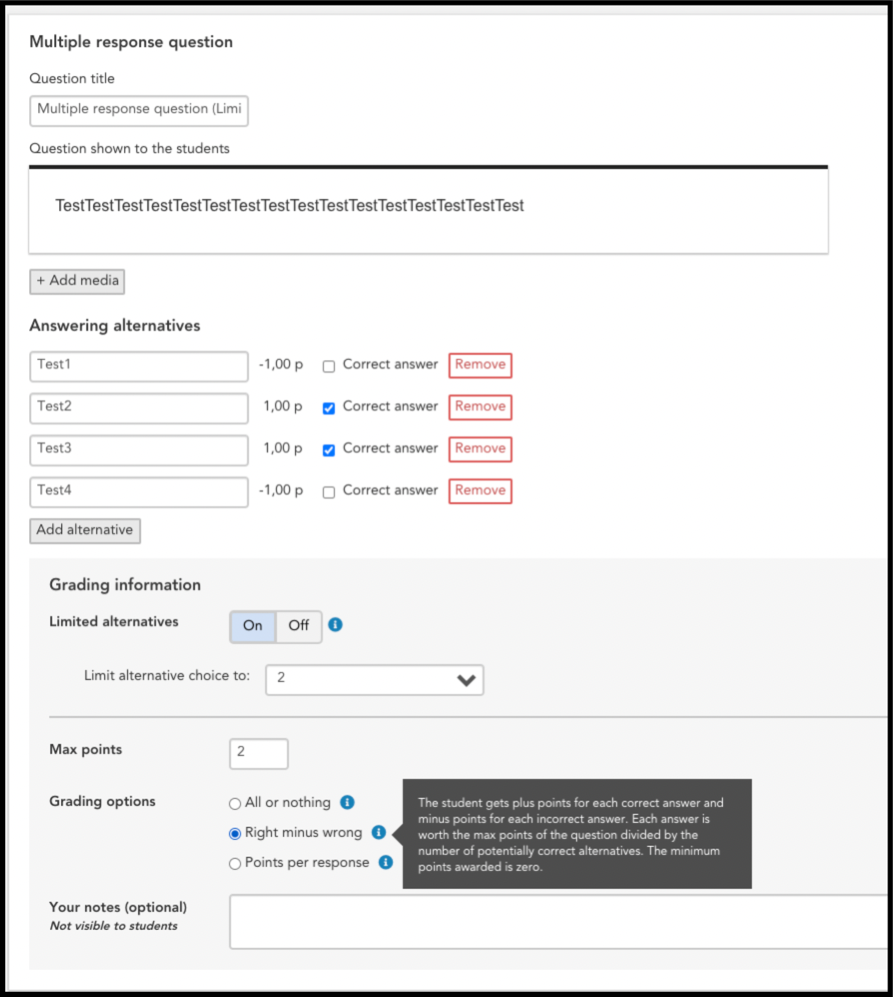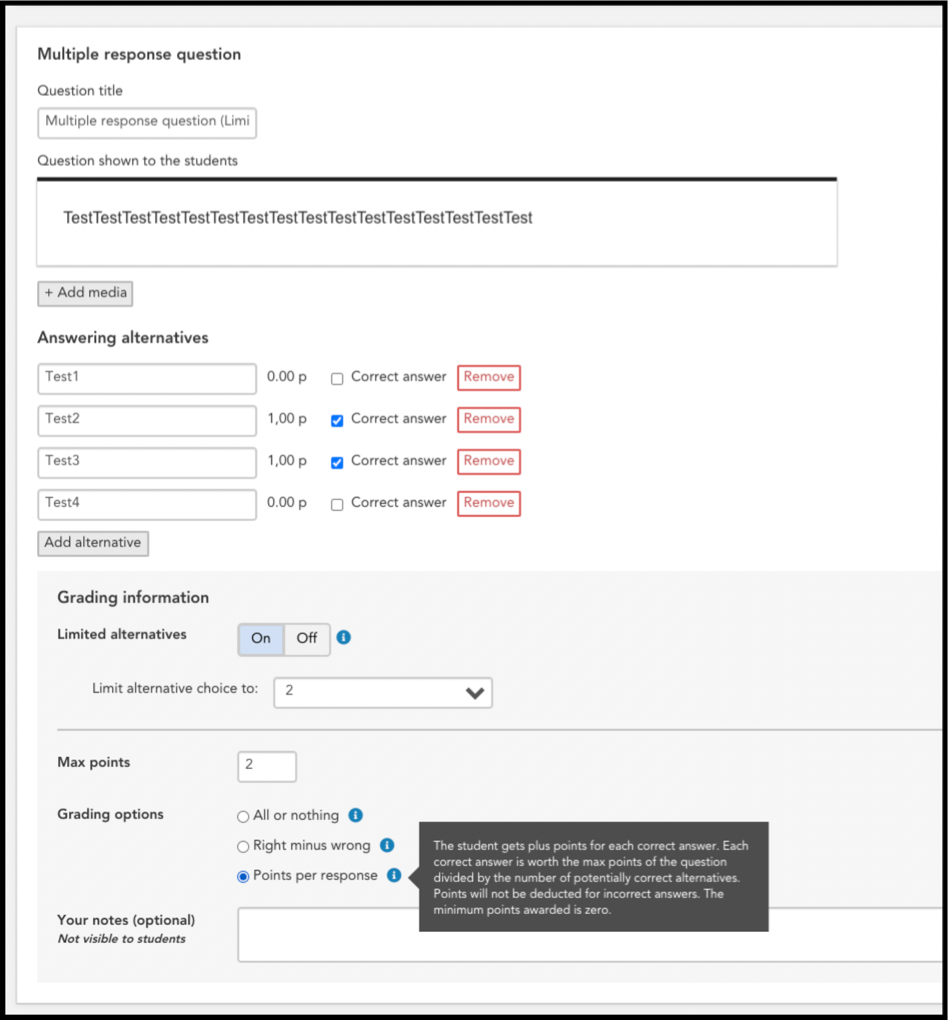Follow

# Multiple response questions

## What is the multiple response questions in Digiexam?

There are 2 types of multiple choice questions, "Multiple Choice Question" and "Multiple Response Answers". Multiple-choice questions in Digiexam are answered by choosing one or more answer options. The multiple response question allows numerous answer alternatives. This should be used when a question has one or more answers that are correct.

When using score based grading, multiple response questions are automatically graded. When using a grading rubric, the multiple response questions are not automatically graded.

### Multiple choice question

In the "Multiple choice question" question type, the student can only choose one correct answer, just as one can only choose one correct answer in the test template.Figure 1Figure 2

### Multiple response question

The question type "Multiple response question" means that the student can choose as many correct answers as they choose in the exam template. It is a crossword question with two or more options where one or more correct answers can be found. These questions are corrected automatically. With this, you can also limit how many boxes the students can choose.

## Grading options for "Multiple response question"

There are three grading options for multiple response questions:

The difference between "Multiple choices" and "Multiple response question" can be seen further down in the image example, where it can be seen that on "Multiple choices" only one option can be selected, while on "Multiple response question" you decide in the test template how many can be checked in.

### All or Nothing

The student must check all correct alternatives to get the max points. If the student misses any of the correct alternatives, or checks any of the incorrect ones, the student is awarded zero points.

In figure 2 below there is an example of a multiple response question in the edit view of an exam with the grading option All or nothing activated.Figure 3

The examples below are based on the question in figure 3.

• Example 1: Student 1 selects Physics and Chemistry = 2 points
• Example 2: Student 2 selects Physics and Literature = 0 points
• Example 3: Student 3 selects Literature and Medicine = 0 points
• Example 4: Student 4 selects Physics = 0 points

### Right Minus Wrong

The student gets plus points for each correct alternative and a minus score for each incorrect alternative. Each alternative is worth the maximum score for the question divided by the number of correct alternatives. The lowest score awarded is zero, so it is not possible to receive minus points (for example -1) for a question.

Figure 3 below shows an example of a multiple response question that is added to the edit view for an exam with the grading option Right minus wrong.Figure 4

The examples below are based on the question in Figure 4.

• Example 1: Student 1 selects Stockholm, London and Copenhagen = 3 points
• Example 2: Student 2 selects Stockholm = 1 point
• Example 3: Student 3 selects Stockholm and London = 2 points
• Example 4: Student 4 selects Stockholm, London and Milan = 2 points
• Example 5: Student 5 selects all options = 0 points
• Example 6: Student 6 selects no options = 0 points
• Example 7: Student 7 selects Milan, Barcelona and Munich = 0 points

### Points per response

The student receives points for the correct answers only. The students do not receive deduction if they choose the wrong alternatives, and only gets points for the correctly chosen alternatives. In order not to give students the opportunity to choose all options and still get the maximum amount of points on the question, there is a function called Limited alternatives, where you can limit the student to a certain number of alternatives they can choose on that question.

Below is an example of a multiple-choice question that is added to the edit view for an exam with the grading option Points per Answer.Figure 5

Note! In this example, the student is limited to max 3 alternatives.

• Example 1: Student 1 chooses Italy, Czechia and Luxembourg = 3 points
• Example 2: Student 2 chooses Chile, Czechia and Malaysia = 1 point
• Example 3: Student 3 chooses Chile and Malaysia = 0 points
• Example 4: Student 4 chooses no alternatives = o points

## Multiple response- and choice question from a student's perspective

The student will see the same multiple response question structure whether the grading type is "All or nothing" or "Right minus error". See examples of multiple response questions when the student answers them in Figures 6 and 7 below.Figure 6Figure 7